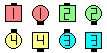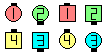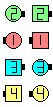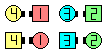Definitions of Square Dance Calls and Concepts
Central Concept [C3B]

Index -->  Plus  |  A1  |  A2  |  C1  |  C2  |  C3A  |  C3B  |  C4  |  NOL  |
Definitions (Text Only) -->  Plus  |  A1  |  A2  |  C1  |  C2  |  C3A  |  C3B  |  C4  |  NOL  |
 Find call:

 \$B8@8l(BDo the Centers part of the anything call. EN: 10
anything \$B%3!<%k\$N(B centers \$B\$N%Q!<%H\$r9T\$\$\$^\$9(B. JP: 10

If the starting formation is a 2 x 4 and if the Centers part of the anything call can be done from either a 1 x 4 or a 2 x 2 (e.g., Counter or Detour), then work in the 2 x 2. For this case, the caller should specify in which setup the dancers are to do the call (e.g., Each Wave do a Central Counter). EN: 20
\$B\$b\$7;O\$a\$N(B formation \$B\$,(B 2 x 4 \$B\$G(B, anything \$B%3!<%k\$N(B centers \$B\$N%Q!<%H\$,(B 1 x 4 \$B\$H(B 2 x 2 \$B\$N\$I\$A\$i\$+\$i\$G\$b=PMh\$k\$H\$-(B (\$BNc\$(\$P(B, Counter \$B\$d(B Detour) \$B\$O(B, 2 x 2 \$B\$GF0\$-\$^\$9(B. \$B\$3\$N>l9g(B, \$B%3!<%i!<\$O%@%s%5!<\$K\$I\$A\$i\$N(B setup \$B\$GF0\$/\$+(B (\$BNc\$(\$P(B, Each Wave do a Central Counter) \$B\$r;X<(\$9\$Y\$-\$G\$9(B. JP: 20

Central Spin The Windmill:Central Spin The Windmill\$B\$NA0(B Swing-Slip\$B\$N8e(B Cast Off 3/4\$B\$N8e(B (\$B=*\$o\$j(B)

Central Ease Off:Central Ease Off\$B\$NA0(B Split Circulate & 1/4 In\$B\$N8e(B (\$B=*\$o\$j(B)

Here is a partial list of calls that might be done Central at C3B: EN: 30
C3B \$B\$G(B Central \$B\$,;H\$(\$k%3!<%k\$N0lIt\$N%j%9%H(B: JP: 30

 Choice Counter Cross Counter Cross Ramble Delight | Dilemma Detour Dodge anything Ease Off Explode The Diamond Fan Chain Thru Hocus Pocus Horseshoe Turn Interlocked Counter Interlocked Little Interlocked Little More Interlocked Rally Interlocked Ramble Little Little More Load The Boat Ramble Rally Regroup Relocate Scramble Spin The Windmill Stampede Team Up Trip The Set TurnStyle

Note that the above list of calls only includes calls in which the Centers remain Centers throughout the entire call. For example, on calls such as Sets In Motion or on Strut Right | Left, the Centers do not end as the Center 4 dancers. On these calls there are normally some outside dancers who end in the Center. Hence, on the Central version of these calls, since there are no outside dancers involved it is ambiguous where the dancers end. Do they leave space for phantoms? EN: 40
\$BCme5-\$N%3!<%k%j%9%H\$K\$O(B, \$B%3!<%k\$N4VCf(B centers \$B\$,(B centers \$B\$K5oB3\$1\$k\$b\$N\$@\$1\$r:\\$;\$F\$\$\$^\$9(B. \$BNc\$(\$P(B, Sets In Motion \$B\$d(B Strut Right | Left \$B\$G\$O(B, centers \$B\$O(B center \$B\$N(B 4 \$B?M\$G\$O=*\$o\$j\$^\$;\$s(B. \$B\$3\$l\$i\$N%3!<%k\$G\$O(B, \$BIaDL(B outside \$B\$N\$"\$k?M\$,(B center \$B\$G=*\$o\$j\$^\$9(B. \$B\$7\$?\$,\$C\$F(B, \$B\$3\$l\$i\$N%3!<%k\$N(B Central \$B\$G\$O(B, \$B\$I\$3\$G=*\$o\$k\$+\$,\$"\$\$\$^\$\$\$G\$9(B. Phantom \$B\$N\$?\$a\$K>l=j\$r;D\$7\$^\$9\$+(B? JP: 40Choreography for Central ConceptComments? Questions? Suggestions?